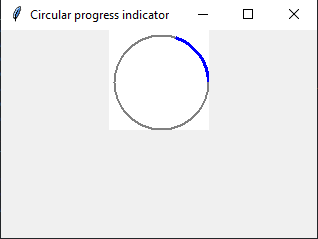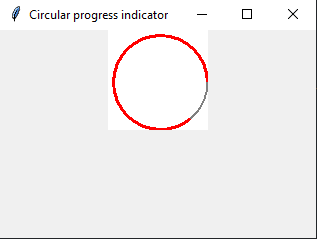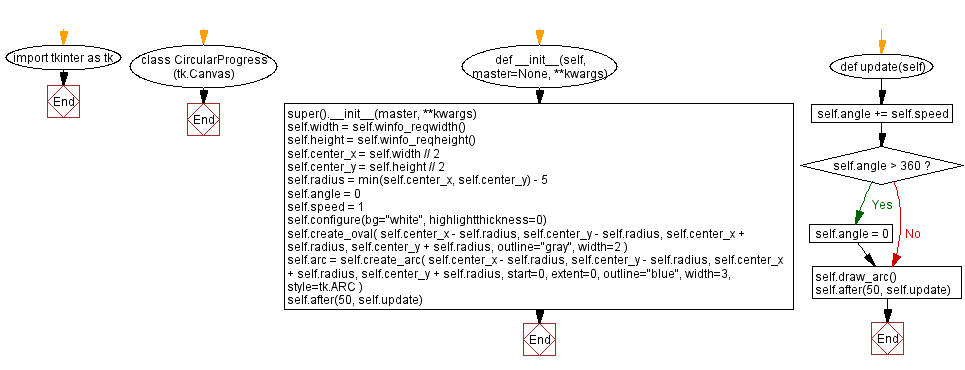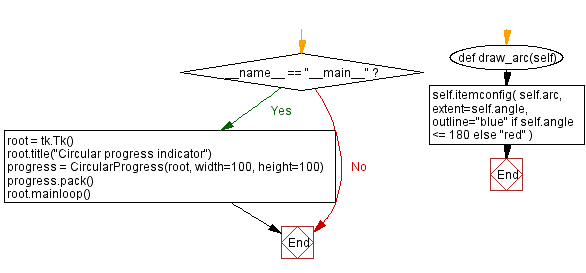﻿ Creating a circular progress indicator in Python with Tkinter

# Creating a circular progress indicator in Python with Tkinter

## Python Tkinter Custom Widgets and Themes: Exercise-11 with Solution

Write a Python program that designs a circular progress indicator widget with customizable colors and animation using Tkinter.

Sample Solution:

Python Code:

``````import tkinter as tk

class CircularProgress(tk.Canvas):
def __init__(self, master=None, **kwargs):
super().__init__(master, **kwargs)
self.width = self.winfo_reqwidth()
self.height = self.winfo_reqheight()
self.center_x = self.width // 2
self.center_y = self.height // 2
self.radius = min(self.center_x, self.center_y) - 5
self.angle = 0
self.speed = 1

self.configure(bg="white", highlightthickness=0)
self.create_oval(
outline="gray", width=2
)

self.arc = self.create_arc(
start=0, extent=0, outline="blue", width=3, style=tk.ARC
)
self.after(50, self.update)

def update(self):
self.angle += self.speed
if self.angle > 360:
self.angle = 0
self.draw_arc()
self.after(50, self.update)

def draw_arc(self):
self.itemconfig(
self.arc,
extent=self.angle,
outline="blue" if self.angle <= 180 else "red"
)

if __name__ == "__main__":
root = tk.Tk()
root.title("Circular progress indicator")
progress = CircularProgress(root, width=100, height=100)
progress.pack()
root.mainloop()
```
```

Explanation:

In the exercise above -

• Create a CircularProgress class that inherits from the tk.Canvas widget.
• Define attributes like width, height, center coordinates, radius, angle, and animation speed.
• Configure the canvas background and create an outer circle to represent the progress indicator.
• The update method increments the angle of the arc and updates its extent to create an animation effect.
• The "draw_arc()" method sets the extent and outline color of the arc based on the current angle.
• In the if name == "__main__": block, we create a Tkinter window and add the 'CircularProgress' widget to it.

Output:

``````
``````

Flowchart:Python Code Editor:

What is the difficulty level of this exercise?

Test your Programming skills with w3resource's quiz.

﻿

## Python: Tips of the Day

Summing a sequence of numbers (calculating the sum of zero to ten with skips):

```>>> l = range(0,10,2)
>>> sum(l)
20
```

We are closing our Disqus commenting system for some maintenanace issues. You may write to us at reach[at]yahoo[dot]com or visit us at Facebook